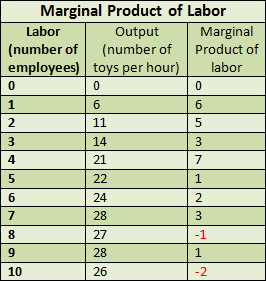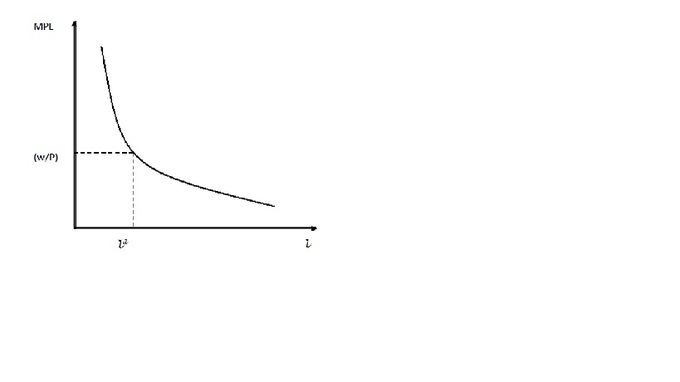Marginal Product of Labor (Physical)

The marginal product of labor is the change in output that results from employing an added unit of labor.

Learning Objectives

Define the marginal product of labor

Key Takeaways

Key Points

• The marginal product of labor is not always equivalent to the output directly produced by that added unit of labor.
• When production is discrete, we can define the marginal product of labor (MPL) as ΔY/ΔL.
• When production is continuous, the MPL is the first derivative of the production function in terms of L.
• Graphically, the MPL is the slope of the production function.
• The law of diminishing marginal returns ensures that in most industries, the MPL will eventually be decreasing.

Key Terms

• returns to scale: A term referring to changes in output resulting from a proportional change in all inputs (where all inputs increase by a constant factor).
• marginal product: The extra output that can be produced by using one more unit of the input.

In economics, the marginal product of labor (MPL) is the change in output that results from employing an added unit of labor. This is not always equivalent to the output directly produced by that added unit of labor; for example, employing an additional cook at a restaurant may make the other cooks more efficient by allowing more specialization of tasks, creating a marginal product that is greater than that produced directly by the new employee. Conversely, hiring an additional worker onto an already crowded factory floor may make the other employees less productive, leading to a marginal product that is lower than the work done by the additional employee.

When production is discrete, we can define the marginal product of labor as ΔY/ΔL where Y is output. If a factory that is initially producing 100 widgets hires another employee and is then able to produce 106 widgets, the MPL is simply six. When production is continuous, the MPL is the first derivative of the production function in terms of L. Graphically, the MPL is the slope of the production function.

gives another example of marginal product of labor. The second column shows total production with different quantities of labor, while the third column shows the increase (or decrease) as labor is added to the production process.Marginal Product of Labor: This table shows hypothetical returns and marginal product of labor. Note that in reality this firm would never hire more than seven employees, since a negative marginal product is bad for the firm regardless of the wage rate.

The law of diminishing marginal returns ensures that in most industries, the MPL will eventually be decreasing. The law states that “as units of one input are added (with all other inputs held constant) a point will be reached where the resulting additions to output will begin to decrease; that is marginal product will decline.” The law of diminishing marginal returns applies regardless of whether the production function exhibits increasing, decreasing or constant returns to scale. The key factor is that the variable input is being changed while all other factors of production are being held constant. Under such circumstances diminishing marginal returns are inevitable at some level of production.

Marginal Product of Labor (Revenue)

The marginal revenue product of labor is the change in revenue that results from employing an additional unit of labor.

Learning Objectives

Define the marginal product of labor under the marginal revenue productivity theory of wages

Key Takeaways

Key Points

• The marginal revenue product of a worker is equal to the product of the marginal product of labor (MP:) and the marginal revenue (MR) of output.
• The marginal revenue productivity theory states that a profit maximizing firm will hire workers up to the point where the marginal revenue product is equal to the wage rate.
• The change in output from hiring one more employee is not limited to that directly attributable to the additional worker.

Key Terms

• marginal product: The extra output that can be produced by using one more unit of the input.
• diminishing marginal returns: The decrease in the per-unit output of a production process as the amount of a single factor of production is increased.

The marginal revenue product of labor (MRPL) is the change in revenue that results from employing an additional unit of labor, holding all other inputs constant. The marginal revenue product of a worker is equal to the product of the marginal product of labor (MPL) and the marginal revenue (MR) of output, given by MR×MP: = MRPL. This can be used to determine the optimal number of workers to employ at an exogenously determined market wage rate. Theory states that a profit maximizing firm will hire workers up to the point where the marginal revenue product is equal to the wage rate, because it is not efficient for a firm to pay its workers more than it will earn in revenues from their labor.

For example, if a firm can sell t-shirts for $10 each and the wage rate is$20/hour, the firm will continue to hire workers until the marginal product of an additional hour of work is two t-shirts. If the MPL is three t-shirts the first will hire more workers until the MPL reaches two; if the MPL is one t-shirt then the firm will remove workers until the MPL reaches two.

Let TR=Total Revenue; L=Labor; Q=Quantity. Mathematically:

• MRPL= ∆TR/∆L
• MR = ∆TR/∆Q
• MPL = ∆Q/∆L
• MR x MPL = (∆TR/∆Q) x (∆Q/∆L) = ∆TR/∆L

Note that the change in output is not limited to that directly attributable to the additional worker. Assuming that the firm is operating with diminishing marginal returns then the addition of an extra worker reduces the average productivity of every other worker (and every other worker affects the marginal productivity of the additional worker) – in other words, everybody is getting in each other’s way.

Because the MRPL is equal to the marginal product of labor times the price of output, any variable that affects either MPL or price will affect the MRPL. For example, changes in technology or the quantity of other inputs will change the marginal product of labor, and changes in the product demand or changes in the price of complements or substitutes will affect the price of output. These will all cause shifts in the MRPL.

Deriving the Labor Demand Curve

Firms will demand labor until the marginal revenue product of labor is equal to the wage rate.

Learning Objectives

Explain how a company uses marginal revenue product in hiring decisions

Key Takeaways

Key Points

• The marginal revenue product of labor (MRPL) is the additional amount of revenue a firm can generate by hiring one additional employee. It is found by multiplying the marginal product of labor by the price of output.
• Firms will demand labor until the MRPL equals the wage rate.
• The demand curve for labor can be shifted by shifted by changes in the productivity of labor, the relative price of labor, or the price of the output.
• It will also change as a result of a change in technology, a change in the price of the good being produced, or a change in the number of firms hiring the labor.

Key Terms

• marginal revenue product: The change in total revenue earned by a firm that results from employing one more unit of labor.
• factor of production: A resource employed to produce goods and services, such as labor, land, and capital.

Firms demand labor and an input to production. The cost of labor to a firm is called the wage rate. This can be thought of as the firm’s marginal cost. The additional revenue generated by hiring one more unit of labor is the marginal revenue product of labor (MRPL). This can be thought of as the marginal benefit.

The marginal revenue product of labor (MRPL) is the additional amount of revenue a firm can generate by hiring one additional employee. It is found by multiplying the marginal product of labor (MPL) – the amount of additional output one additional worker can generate – by the price of output. If an employee of a customer support call center can take eight calls an hour (the MPL) and each call earns the company $3, then the MRPL is$24.

We can use the MRPL curve to determine the quantity of labor a company will hire. Suppose workers are available at an hourly rate of $10. The amount a factor adds to a firm’s total cost per period is the marginal cost of that factor, so in this case the marginal cost of labor is$10. Firms maximize profit when marginal costs equal marginal revenues, and in the labor market this means that firms will hire more employees until the wage rate (marginal cost of labor) equals the MRPL. At a price of $10, the company will hire workers until the last worker hired gives a marginal revenue product of$10.Marginal Product of Labor: The MPL falls as the amount of labor employed increases. The optimum demand for labor falls where the real wage rate (w/P) is equal to the MPL.

Thus, the downward-sloping portion of the marginal revenue product curve shows the number of employees a company will hire at each price (wage), so we can interpret this part of the curve as the firm’s demand for labor. As with other demand curves, the market demand curve for labor is the sum of all firm’s individual demand curves.

Shifting the Demand for Labor

There are three main reasons why the demand curve for labor may shift:

1. Changes to the marginal productivity of labor: Technology, for instance, may increase the marginal productivity of labor, shifting the demand curve to the right. For example, computer technology has increased the productivity (marginal product) of many types of workers. This has led to an increase in the marginal revenue product of labor for these jobs, shifting firms’ demand for labor to the right. This both increases the number of employed workers and increases the wage rate.
2. The prices of other factors of production: The change in the relative price of labor will increase or decrease demand for labor. For example, is capital becomes more expensive relative to labor, the demand for labor will increase as firms seek to substitute labor for capital.
3. The price of the firm’s output: Since the price of the output is a component of MRPL, changes will shift the demand curve for labor. If the price that a firm can charge for its output increases, for example, the MRPL will increase. This is reflected in an outward shift of the demand for labor.